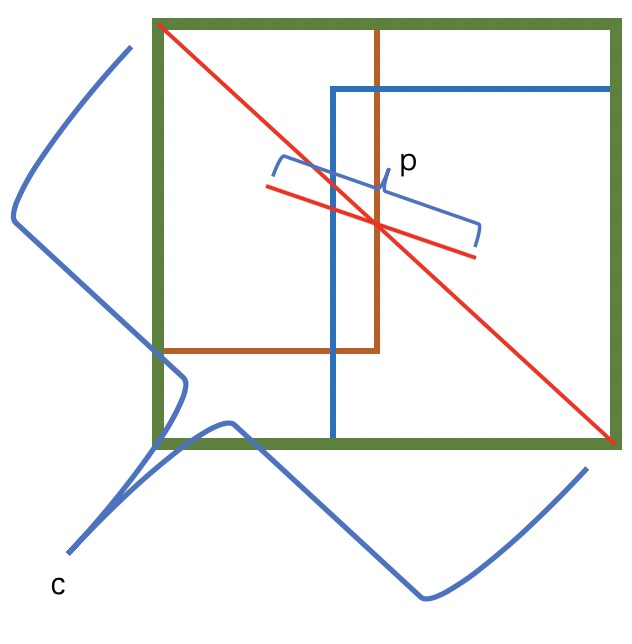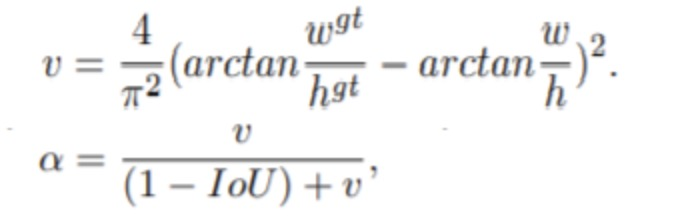# 目标检测IOU评价指标汇总: GIOU, DIOU, CIOU

## GIOU

IOU只考虑物体重叠部分，同时不能作为直接作为损失进行优化, GIOU 在IOU基础上既关注重叠部分也关注不重叠部分

$$GIOU = IOU - \frac{|A_c - U |}{|A_c|}$$

$A_c$ 为两个框的最小闭包矩形, 如下图中的绿色框. $U$ 为两个框的并，即为下图的蓝色区域，GIOU通过计算空白区域的占比考虑相交的方式。## DIOU（Distance-IOU）

$$DIOU=IOU - \frac{p ^2 (b, b ^{gt})}{c^2}$$

$b$ 代表预测框与GT框的中心点，$p ^2$ 表示计算二者的欧式距离， $c$代表的是能够同时包含预测框和真实框的最小闭包区域的对角线距离.• DIoU loss可以直接最小化两个目标框的距离，因此比GIoU loss收敛快得多
• 对于包含两个框在水平方向和垂直方向上这种情况，DIoU损失可以使回归非常快，而GIoU损失几乎退化为IoU损失

## CIOU

GIOU考虑了重叠与非重叠区域，DIOU在GIOU基础上考虑了中心点距离，CIOU又引进了长宽比

$$CIOU=IOU - \frac{p ^2 (b, b ^{gt})}{c^2} - \alpha v$$